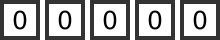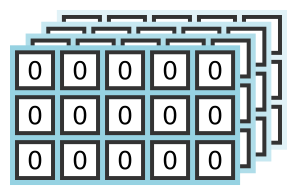# Python informer

Improve your Python coding skills

## Anatomy of a numpy array

Numpy arrays come is various types, shapes and sizes. In this article will look at different array parameters, and learn the correct terms used by numpy.

## Rank

The rank of an array is simply the number of axes (or dimensions) it has.

A simple list has rank 1:A 2 dimensional array (sometimes called a matrix) has rank 2:A 3 dimensional array has rank 3. It is shown here as a stack of matricesIt gets difficult to draw arrays with more than 3 dimensions, but numpy allows you to have as many dimensions as you want.

## Shape

The shape of gives the length of the array in each dimension. It is usually represented as a tuple. For a given array, the number of elements in the shape tuple will be equal to the rank of the array.

Our rank 1 array above has 5 elements, so its shape is the tuple (5,).

The rank 2 array has 3 rows and 5 columns, so its shapes is (3, 5). By convention, we take the matrix shape to be rows first, then columns.

The rank 3 array has 4 planes, each containing 3 rows and 5 columns, so its shapes is (4, 3, 5). By convention, we take the matrix shape to be planes first, then rows, then columns.

The shape of an array can be found using the ndim attribute.

## Size

The size of an array is simply the total number of elements. It is found by multiplying together all the elements of the shape.

Our rank 1 array above has 5 elements, so its size is 5.

The rank 2 array has shape 3 by 5, so its size is 15 (there are 15 elements in total).

The rank 3 array has shape 4 by 3 by 5, so its size is 60 (there are 60 elements in total).

The size of an array can be found using the size attribute.

## Data type

Unlike a Python list, numpy arrays are made up of primitive data types. These are data types, typically ints or floats, that are stored directly in memory without any extra information.

For example, data type numpy.int32 is a 32 bit integer that occupies exactly 4 byte (32 bits) of memory.

Numpy offers different sizes of ints and floats, which you can choose to match the data you are dealing with. For example, if you are processing image data, each pixel’s red, green and blue values will usually be represented as an 8 bit integer value. You could store the image in a numpy array of int32 values, but that would use 4 times as much memory as is necessary, so you would be better off using the int8 data type.

The data type of an array can be found using the dtype attribute.

## Other array parameters

You can find the size of an element in an array using the itemsize attribute. The element size is determined by the data type, so for example an int32 array will have an item size if 4 (32 bits divided by 8 gives 4 bytes). An int8 array with have an item size of 1, etc.

You can also get the memory address of the actual array data, using the data attribute. That isn’t particularly useful if you are writing a pure Python program, because Python can’t directly access memory locations. It can be useful if you are calling other low level libraries that have Python bindings and can accept memory pointers as parameters.

## Example

Here is the code to create a 3 by 5 array of zeros, and check its parameters.

import numpy as np

a2 = np.zeros((3, 5))

print(a2.ndim)      # 2
print(a2.shape)     # (3, 5)
print(a2.size)      # 5
print(a2.dtype)     # float64
print(a2.itemsize)  # 8 (float64 is an 8 byte quantity)
print(a2.data)      # <memory at XXXX>